Figures index

Shalaan A. M. Alshammari, Mostafa M. Makrahy, Nouby M. Ghazaly

Journal of Mechanical Design and Vibration. 2019, 7(1), 21-26 doi:10.12691/jmdv-7-1-3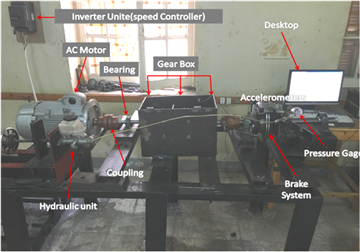• Figure 1. Gearbox fault diagnosis test rig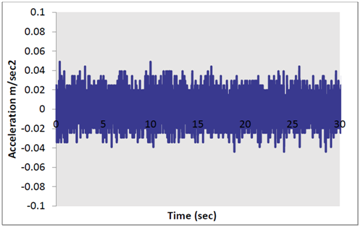• Figure 2. Time Domain Vibration Signal of the Normal Gear at 100 rpm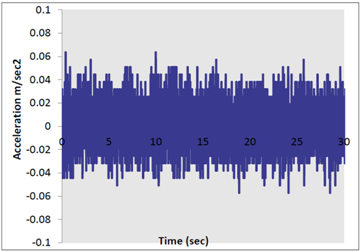• Figure 3. Time Domain Vibration Signal of the Normal Gear at 200 rpm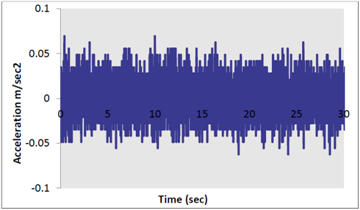• Figure 4. Time Domain Vibration Signal of the Normal Gear at 300 rpm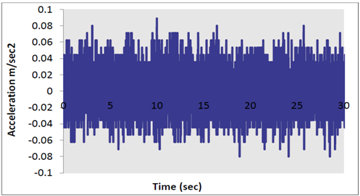• Figure 5. Time Domain Vibration Signal of the Normal Gear at 400 rpm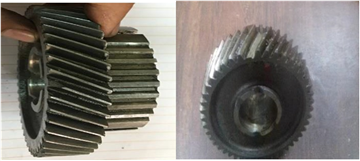• Figure 6. Proposed helical gear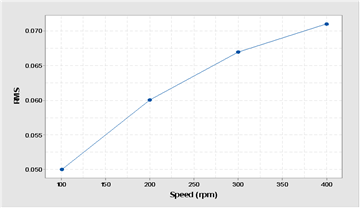• Figure 7. RMS of the Normal Gear with Different Speeds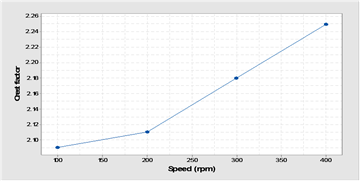• Figure 8. Crest Factor of the Normal Gear with Different Speeds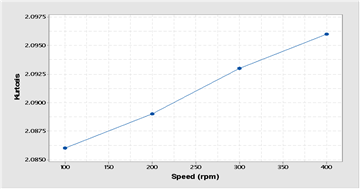• Figure 9. Kurtosis of the Normal Gear with Different Speeds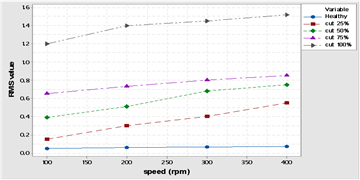• Figure 10. RMS Vibration Measurement Tooth Breakage Defect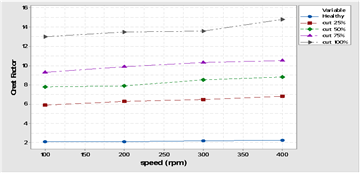• Figure 11. Crest Factor Vibration Measurement Tooth Breakage Defect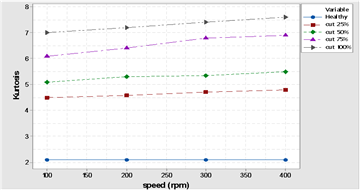• Figure 12. Kurtosis Vibration Measurement Tooth Breakage Defect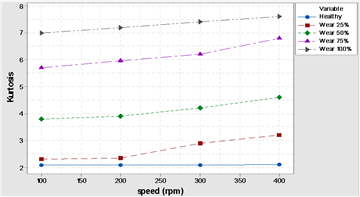• Figure 15. Kurtosis Vibration Measurement Tooth Wear